# oscillator

Also found in: Dictionary, Thesaurus, Medical, Legal, Financial, Acronyms, Idioms, Wikipedia.

## oscillator

[′äs·ə‚lād·ər]
(electronics)
An electronic circuit that converts energy from a direct-current source to a periodically varying electric output.
The stage of a superheterodyne receiver that generates a radio-frequency signal of the correct frequency to mix with the incoming signal and produce the intermediate-frequency value of the receiver.
The stage of a transmitter that generates the carrier frequency of the station or some fraction of the carrier frequency.
(physics)
Any device (mechanical or electrical) which, in the absence of external forces, can have a periodic back- and-forth motion, the frequency determined by the properties of the oscillator.

## Oscillator

a physical system that performs oscillations. The term “oscillator” is used for any system that can be described by variables that change periodically with time.

Classical oscillator. The classical oscillator is a mechanical system that vibrates around a fixed equilibrium point. In the equilibrium position the potential energy U of the system is at a minimum. If the deviation x from this position is small, then the power-series expansion of U(x) is approximated by the expression kx2/2, where K is a constant, and the quasi-elastic force F = – ∂ U/∂x = – kx (seeQUASI-ELASTIC FORCE). Such oscillators are called harmonic oscillators and their motion is described by the linear equation mẍ = –kx, which has a solution of the form x = A sin (ωt + ϕ), where m is the mass of the oscillator, ω is the angular frequency (which equals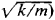, A is the vibration amplitude, ϕ is the initial phase, and t is time. The total energy E of a harmonic oscillator is given by mω2A2/2, which is the sum of the kinetic energy T and potential energy U. These energies are periodically changing with opposite phases, and the total energy E = T + U is independent of time.

When the deviation x cannot be regarded as small, it is necessary to take into account higher-order terms in the expansion of U(x): the equation of motion becomes nonlinear, and the oscillator is called anharmonic.

The principle of an oscillator can also be applied to non-mechanical oscillating systems, for example, in electromagnet-ism, acoustics, and gravitational theory. The most frequently encountered application is the electrical oscillator—an oscillating circuit with inductance and capacitance. The oscillations of the electric and magnetic field intensities in a plane electromagnetic wave can also be described using the principle of oscillators.

Quantum oscillator. The problem of the linear harmonic oscillator with one degree of freedom in quantum mechanics is solved by the Schrödinger equation, in which the potential energy U is assumed to be equal to kx2/2. A solution exists for only a discrete set of energy values: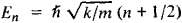where h is Planck’s constant and n is zero or any positive integer. An important feature of the energy spectrum for this oscillator is that the levels of the energies En are equally spaced. Because the selection rules permit transitions only between adjacent energy levels, the radiation of a quantum oscillator has a single frequency, ω—although a quantum oscillator has a set of natural frequencies ωn = En/ℏ. This agrees with the classical case: ω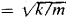. Unlike the case that holds for the classical oscillator, the lowest possible energy level (when n = 0) of the quantum oscillator is not equal to zero but to ℏω/2 (the condition that corresponds to zero energy).

The oscillator principle plays an important role in solid-state theory and in the theories of electromagnetic radiation and molecular vibrational spectra.

### REFERENCES

Landau, L. D., and E. M. Lifshits. Mekhanika: Elektrodinamika, ch. 5. (Kratkii kurs teoreticheskoi fiziki, book 1.) Moscow, 1969.
Landau, L. D., and E. M. Lifshits. Teoriia polia, 5th ed. (Teoreticheskaia fizika, vol. 2.) Moscow, 1967.
Landau, L. D., and E. M. Lifshits. Kvantovaia mekhanika. (Teoreticheskaia fizika, vol. 3.) Moscow, 1963.
Leontovich, M. A. Statisticheskaia fizika. Moscow-Leningrad, 1944.

V. P. PAVLOV

## Oscillator

An electronic circuit that generates a periodic output, often a sinusoid or a square wave. Oscillators have a wide range of applications in electronic circuits: they are used, for example, to produce the so-called clock signals that synchronize the internal operations of all computers; they produce and decode radio signals; they produce the scanning signals for television tubes; they keep time in electronic wristwatches; and they can be used to convert signals from transducers into a readily transmitted form.

Oscillators may be constructed in many ways, but they always contain certain types of elements. They need a power supply, a frequency-determining element or circuit, a positive-feedback circuit or device (to prevent a zero output), and a nonlinearity (to define the output-signal amplitude). Different choices for these elements give different oscillator circuits with different properties and applications.

Oscillators are broadly divided into relaxation and quasilinear classes. Relaxation oscillators use strong nonlinearities, such as switching elements, and their internal signals tend to have sharp edges and sudden changes in slope; often these signals are square waves, trapezoids, or triangle waves. The quasilinear oscillators, on the other hand, tend to contain smooth sinusoidal signals because they regulate amplitude with weak nonlinearities. The type of signal appearing internally does not always determine the application, since it is possible to convert between sine and square waves. Relaxation oscillators are often simpler to design and more flexible, while the nearly linear types dominate when precise control of frequency is important.

#### Relaxation oscillators

Illustration a shows a simple operational-amplifier based relaxation oscillator. This circuit can be understood in a number of ways (for example, as a negative-resistance circuit), but its operation can be followed by studying the signals at its nodes (illus. b). The two resistors, labeled r, provide a positive-feedback path that forces the amplifier output to saturate at the largest possible (either positive or negative) output voltage. If v+, for example, is initially slightly greater than v-, then the amplifier action increases vo, which in turn further increases v+ through the two resistors labelled r. This loop continues to operate, increasing vo until the operational amplifier saturates at some value Vmax. [An operational amplifier ideally follows Eq.

(1)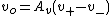(1), where Av is very large, but is restricted to output levels &verbar;vo&verbar; ≤ Vmax.] For the purposes of analyzing the circuit, the waveforms in the illustration have been drawn with the assumption that this mechanism has already operated at time 0 and that the initial charge on the capacitor is zero. See Amplifier, Operational amplifier

Capacitor C will now slowly change from vo through resistor R, toward Vmax, according to Eq. (2). (2)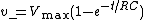Up until time t1, this process continues without any change in the amplifier's output because v+ > v-, and so vo = Vmax. At t1, however, v+ = v- and vo will start to decrease. This causes v+ to drop, and the positive-feedback action now drives the amplifier output negative until vo = -Vmax. Capacitor C now discharges exponentially toward the new output voltage until once again, at time t2, v+ = v-, and the process starts again. The period of oscillation for this circuit is 2RC ln 3.

The basic elements of an oscillator that were mentioned above are all clearly visible in this circuit. Two direct-current power supplies are implicit in the diagram (the operational amplifier will not work without them), the RC circuit sets frequency, there is a resistive positive-feedback path that makes the mathematical possibility vo(t) = 0 unstable, and the saturation behavior of the amplifier sets the amplitude of oscillation at the output to ±Vmax.

Relaxation oscillators that have a low duty cycle—that is, produce output pulses whose durations are a small fraction of the overall period—are sometimes called blocking oscillators because their operation is characterized by an “on” transient that “blocks” itself, followed by a recovery period.

Inverters (digital circuits that invert a logic signal, so that a 0 at the input produces a 1 at the output, and vice versa) are essentially voltage amplifiers and can be used to make relaxation oscillators in a number of ways. A circuit related to that of the illustration uses a loop of two inverters and a capacitor C to provide positive feedback, with a resistor R in parallel with one of the inverters to provide an RC charging time to set frequency. This circuit is commonly given as a simple example, but there are a number of problems with using it, such as that the input voltage to the first gate sometimes exceeds the specified limits for practical gates. A more practical digital relaxation oscillator, called a ring oscillator, consists simply of a ring containing an odd number N (greater than 1) of inverters. See Logic circuits

#### Sine-wave oscillators

Oscillators in the second major class have their oscillation frequency set by a linear circuit, and their amplitudes set by a weak nonlinearity.

A simple example of a suitable linear circuit is a two-component loop consisting of an ideal inductor [whose voltage is given by Eq. (3),

(3)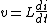where i is its current] and a capacitor [whose current is given by Eq. (4)],
(4)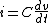connected in parallel. These are said to be linear elements because, in a sense, output is directly proportional to input, for example, doubling the voltage v across a capacitor also doubles dv/dt and therefore doubles i. The overall differential equation for a capacitor-inductor loop can be written as Eq. (5). (5)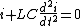Mathematically this has solutions of the form of Eq. (6),
(6)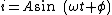where &ohgr; = 1/LC [which means that the circuit oscillates at a frequency 1/(2&pgr;LC)] and A and &phgr; are undefined. They are undefined precisely because the elements in the circuit are linear and do not vary with time: any solution (possible behavior) to the equation can be scaled arbitrarily or time-shifted arbitrarily to give another. Practically, A and &phgr; are determined by weak nonlinearities in a circuit.

Equation (5) is a good first approximation to the equation describing a pendulum, and so has a long history as an accurate timekeeper. Its value as an oscillator comes from Galileo's original observation that the frequency of oscillation (&ohgr;/2&pgr;) is independent of the amplitude A. This contrasts sharply with the case of the relaxation oscillator, where any drift in the amplitude (resulting from a threshold shift in a comparator, for instance) can translate directly into a change of frequency. Equation (5) also fundamentally describes the operation of the quartz crystal that has replaced the pendulum as a timekeeper; the physical resonance of the crystal occurs at a time constant defined by its spring constant and its mass.

#### Frequency locking

If an external signal is injected into an oscillator, the natural frequency of oscillation may be affected. If the external signal is periodic, oscillation may lock to the external frequency, a multiple of it, or a submultiple of it, or exhibit an irregular behavior known as chaos.

This locking behavior occurs in all oscillators, sometimes corrupting intended behavior (as when an oscillator locks unintentionally to a harmonic of the power-line frequency) and sometimes by design. An important example of an oscillator that exploits this locking principle is the human heart. Small portions of heart muscle act as relaxation oscillators. They contract, incidentally producing an output voltage that is coupled to their neighbors. For a short time the muscle then recovers from the contraction. As it recovers, it begins to become sensitive to externally applied voltages that can trigger it to contract again (although it will eventually contract anyway). Each small section of heart muscle is thus an independent oscillator, electrically coupled to its neighbors, but the whole heart is synchronized by the frequency-locking mechanism.

## oscillator

i. An electronic circuit that converts energy from a direct-current source to a periodically varying electric output.
ii. The stage of a superheterodyne receiver that generates a radio-frequency signal of the correct frequency to mix with the incoming carrier signal to produce the intermediate-frequency value of the receiver.
iii. The stage of a transmitter that generates the carrier frequency or some fraction of the carrier frequency.

## oscillator

An electronic circuit used to generate high-frequency pulses. See crystal oscillator, VCO and clock.
References in periodicals archive ?
In addition, an emerging use of MEMS technology is as a replacement for traditional crystal oscillators. Here, we will compare the characteristics and advantages of MEMS vs.
The main idea is to eliminate the disadvantages of symmetrical load differential VCO, and play to their strengths, to design a new extension delay unit configuration, so that a cascade of 3 stages of these units constitute a High Performance Voltage-Controlled oscillator (HPVCO), characterized by a stable full-swing output with a wide range of voltage regulation.
The design of the active frequency stabilization oscillator and the initial experimental verification started from the hypothesis according to which the performances of an oscillator can be significantly enhanced by replacing the varicap diode with a BST capacitor.
In order to validate our theoretical analysis above, we are going to build a model of an effective negative mass system with pure Duffing oscillator in five periods as shown in Figure 4.
Scholl, "Amplitude death in oscillator networks with variable-delay coupling," Physical Review E, vol.
Nevertheless, it should be noted that the Taylor expansion may not be applicable to the PN of a free-running oscillator, whose variance increases linearly with time, after long transmission.) As a result, (30) can be approximated as
The test revealed that the average phase noise spur power due to EMI was lower for the MEMS oscillator than for any of the quartz oscillators in this study.
During a chaotic event the maximum amplitudes of oscillation are far from being symmetrical about the equilibrium position of the oscillator.
(ii) The nonlinear oscillator equation has converted to nonlinear algebraic equation by this approach which can be solved by numerical methods that leads to a better result.
Holzel, "Simple wide-band sine wave quadrature oscillator," IEEE Transactions on Instrumentation and Measurement, vol.
The Effect of the Higher-Order Coupling Term in Inducing the Synchronization of the Inhibitory Neuronal Oscillator Population under Spontaneous Activity.
The next step would be building a larger network of oscillators that could handle graph coloring problems with more objects at play.

Site: Follow: Share:
Open / Close